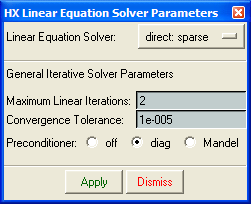﻿ Linear Equation Solver
Manufacturing Solutions

# Linear Equation Solver# Linear Equation SolverSelecting the Iterative Solver option on the Solver menu brings up the Iterative Solver Parameters panel. This panel allows you to define the parameters associated with the iterative solver used to solve the linear system of equations. HyperXtrude allows you to use either a direct solver (Sparse) or an iterative solver (GMRES) to solve the linear system of equations. The convergence behavior of the iterative solver depends on the type of pre-conditioner used. To accelerate the convergence of the linear equation solver, HyperXtrude uses two types of pre-conditioners, diagonal and Mandel. The Maximum Linear Iterations: option denotes the number of GMRES restarts allowed. Convergence Tolerance: denotes the user-specified tolerance for the residual. The value entered in the Sub Iterations: field represents the number of iterations within each restart.The Linear Equation Solver panel